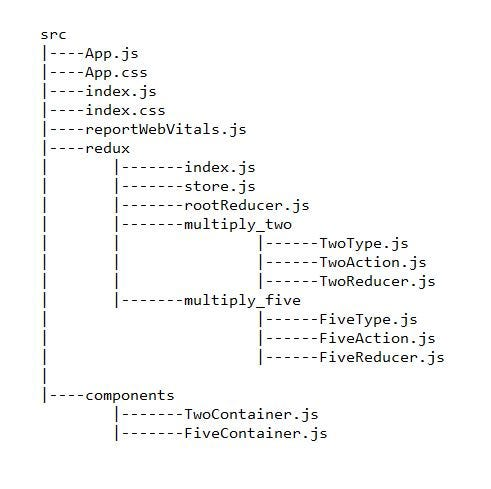# How to Use combineReducers() in Redux and ReactIn this article, we’ll create a simple React application where we’ll use redux for state management. The final look of this application is shown below —

In this exercise, we’ll use `combineReducers()` of the `redux` library which combines multiple reducers into a single reducer.

According to the official documentation, As our application grows and becomes more complex, we can have multiple reducers each managing independent parts of the state. The `combineReducers()` helper function turns an object whose values are different reducing functions into a single reducing function that we can pass to `createStore()`.

### Initial setup

First of all, we have make sure that Node.js is installed in our system. If so, we’ll create a directory with name React_Projects and then, we’ll open the terminal and navigate into that directory. There, we’ll run the following command —

``````npx create-react-app myapp
``````

Then we’ll run the run the following command to install `redux` and `react-redux`.

``````npm install redux react-redux
``````

`redux` is a state management library.

And, `react-redux` helps to connect react with redux.

### Procedure

The `src` directory for this project will look like the following —#### Step 1

First of all, we’ll set up the redux store for our application. For that we’ll create a directory named `redux` inside `src`.

Since, we’ll have two reducers for handling both the states, that’s why we’ll create two directories inside the `redux` directory.

#### Step 2

The first directory inside the `redux` directory will be `multiply_two`. In this directory, there will be three files which are given below —

``````export const MULTIPLY_TWO = "MULTIPLY_TWO";
``````
``````import { MULTIPLY_TWO } from "./TwoType";

export const multiplyTwo = () => {
return {
type: MULTIPLY_TWO,
};
};
``````
``````import { MULTIPLY_TWO } from "./TwoType";

const initialState = {
val_two: 1,
};

const TwoReducer = (state = initialState, action) => {
switch (action.type) {
case MULTIPLY_TWO:
return {
...state,
val_two: state.val_two * 2,
};

default:
return state;
}
};

export default TwoReducer;
``````

#### Step 3

The second directory inside the `redux` directory will be `multiply_five`. In this directory also, there will be three files which are given below —

``````export const MULTIPLY_FIVE = "MULTIPLY_FIVE";
``````
``````import { MULTIPLY_FIVE } from "./FiveType";

export const multiplyFive = () => {
return {
type: MULTIPLY_FIVE,
};
};
``````
``````import { MULTIPLY_FIVE } from "./FiveType";

const initialState = {
val_five: 1,
};

const FiveReducer = (state = initialState, action) => {
switch (action.type) {
case MULTIPLY_FIVE:
return {
...state,
val_five: state.val_five * 5,
};

default:
return state;
}
};

export default FiveReducer;
``````

#### Step 4

Now, we’ll combine both the reducers in `rootReducer.js` file.

``````import { combineReducers } from "redux";
import TwoReducer from "./multiply_two/TwoReducer";
import FiveReducer from "./multiply_five/FiveReducer";

const rootReducer = combineReducers({
two: TwoReducer,
five: FiveReducer,
});

export default rootReducer;
``````

In the above code, the `combineReducers()` method accepts an object whose keys can be any name that we want to provide and the value are the reducer functions.

#### Step 5

Now, we’ll create the redux store in `store.js` file.

``````import { createStore } from "redux";
import rootReducer from "./rootReducer";

const store = createStore(rootReducer);

export default store;
``````

#### Step 6

In this step, we’ll create a file `index.js` which will export both the action creator functions, viz. `multiplyTwo` and `multiplyFive`.

``````export { multiplyTwo } from "./multiply_two/TwoAction";

export { multiplyFive } from "./multiply_five/FiveAction";
``````

#### Step 7

Now, we’ll create a `components` directory inside `src` directory, which will contain two components that will be rendered.

The first component will be `TwoContainer.js`, whose code is given below —

``````import React from "react";
import { connect } from "react-redux";
import { multiplyTwo } from "../redux";

function TwoContainer(props) {
return (
<div className="TwoContainer">
<h1>{props.val_two}</h1>
<h3>Click the button to multiply the above number by 2</h3>
<button onClick={props.multiplyTwo}>Multiply by 2</button>
</div>
);
}

const mapStateToProps = (state) => {
return {
val_two: state.two.val_two,
};
};

const mapDispatchToProps = (dispatch) => {
return {
multiplyTwo: () => dispatch(multiplyTwo()),
};
};

export default connect(mapStateToProps, mapDispatchToProps)(TwoContainer);
``````

The second component will be `FiveContainer.js`, whose code is given below —

``````import React from "react";
import { connect } from "react-redux";
import { multiplyFive } from "../redux";

function FiveContainer(props) {
return (
<div className="FiveContainer">
<h1>{props.val_five}</h1>
<h3>Click the button to multiply the above number by 5</h3>
<button onClick={props.multiplyFive}>Multiply by 5</button>
</div>
);
}

const mapStateToProps = (state) => {
return {
val_five: state.five.val_five,
};
};

const mapDispatchToProps = (dispatch) => {
return {
multiplyFive: () => dispatch(multiplyFive()),
};
};

export default connect(mapStateToProps, mapDispatchToProps)(FiveContainer);
``````

In the above codes, the `mapStateToProps` function has the following statements —

`val_two: state.two.val_two` and `val_five: state.five.val_five`.

Both the above statements are a key-value pair and the values are referring to the state’s keys `two` and `five`, defined in `rootReducer.js`.

#### Step 8

Finally, we’ll modify the `App.js` file into the following —

``````import React from "react";
import { Provider } from "react-redux";
import store from "./redux/store";
import TwoContainer from "./components/TwoContainer";
import FiveContainer from "./components/FiveContainer";
import "./App.css";

function App() {
return (
<Provider store={store}>
<div className="App">
<TwoContainer />
<br />
<FiveContainer />
</div>
</Provider>
);
}

export default App;
``````

Now, our exercise is complete and we can run the development server and check the result.

All the codes are also available in my GitHub repository which can be accessed using the following link —

souvik-pl/react_redux_combineReducers

### References

https://redux.js.org/api/combinereducers

Documentation — Combine Reducers